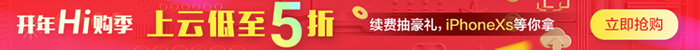#1. 云栖社区>
2. 博客>
3. 正文## 二项分布的期望值 E(n)=np 推导

n表示n次试验，p表示单次试验的成功概率。
E(n)表示n次试验的成功次数的数学期望。

∑k=0,n    C(n,k) * p^k * (1-p)^(n-k) = 1

∑k=0,n   k * P(k)
=
∑k=1,n   k * P(k)

E(n)=np这个公式是如何推导来的呢？

000000
100000
010000
001000
000100
000010
000001
......

n次试验，成功k次的可能性有多少种=C(n,k)=n! / (k!(n-k)!)
n次试验，成功k次的概率=C(n,k) * p^k * (1-p)^(n-k)

∑k=0,n    C(n,k) * p^k * (1-p)^(n-k) = 1

∑k=1,n   k * C(n,k) p^k * (1-p)^(n-k)
=
∑k=1,n   k * (n! / (k!(n-k)!)) p^k * (1-p)^(n-k)
=
∑k=1,n   k * (n! / (k(k-1)!(n-k)!)) p^k * (1-p)^(n-k)
=
∑k=1,n   (n! / ((k-1)!(n-k)!)) p^k * (1-p)^(n-k)
=
∑k=1,n   (n(n-1)! / ((k-1)!(n-k)!)) * p * p^(k-1) * (1-p)^(n-k)
=
np∑k=1,n   ((n-1)! / ((k-1)!(n-k)!)) * p^(k-1) * (1-p)^(n-k)

a=k-1
b=n-1
n-k = (a+1) - (b+1) = b-a

=
np∑a=0,b   ((b)! / ((a)!(b-a)!)) p^(a) * (1-p)^(b-a)

∑k=0,n    C(n,k) * p^k * (1-p)^(n-k) = 1

[参考]
1. 可汗学院公开课：统计学24-二项分布的期望值
http://v.ku6.com/special/show_6598382/L6rDnRd-Ra0eamhycyU8ag...html
【云栖快讯】一站式开发者服务，海量学习资源免费学  详情请点击

+ 关注